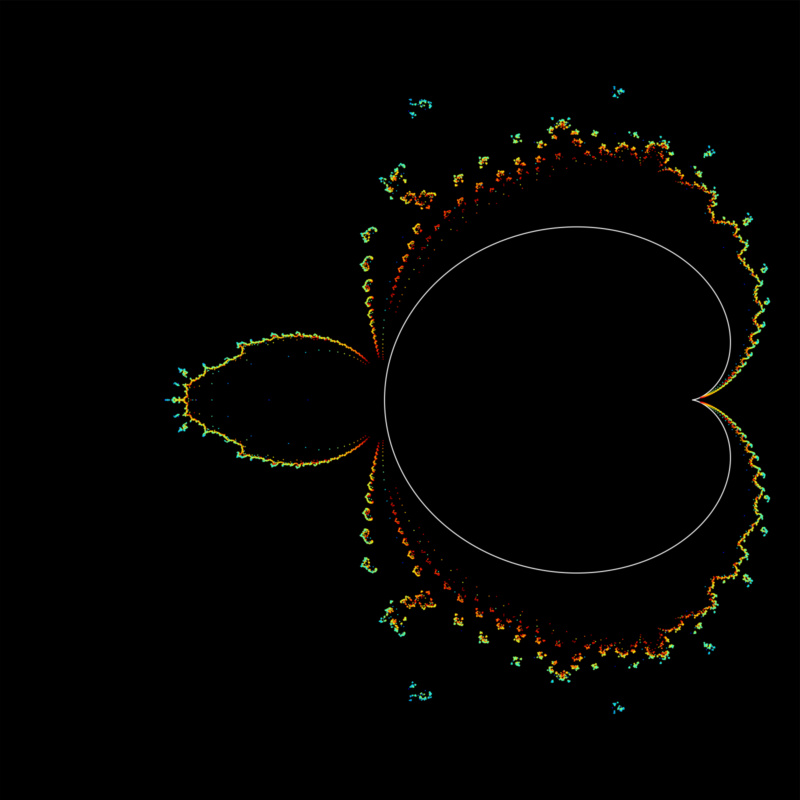# Bohemian MatricesA plot in the complex plane of the roots of the Narayana-Mandelbrot polynomials $r_0(z) = r_1(z) = r_2(z) = r_{n+1}(z) = zr_{n}(z)r_{n-2}(z) + 1$ for degrees 4 through 36. The roots are computed as the eigenvalues of a specialized recursively-constructed, supersparse, upper Hessenberg matrix. Color represents the minimum degree Narayana-Mandelbrot polynomial the root is a solution of. The plot is viewed on [-2-1.25i, 0.5+1.25i]. Plot produced by Eunice Chan. For more information see: A comparison of solution methods for Mandelbrot-like polynomials.